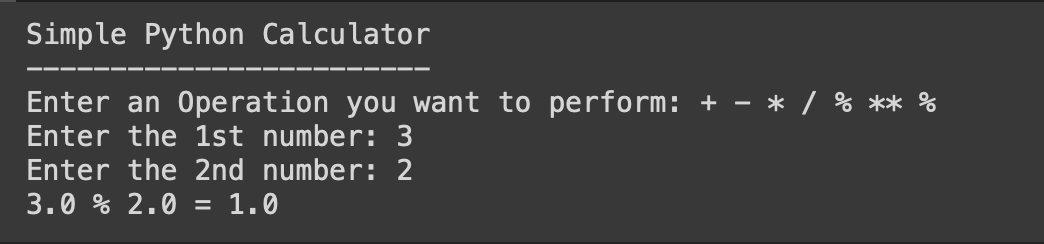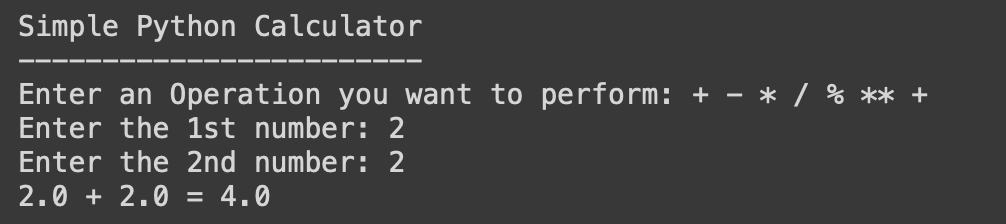# 19: Simple Calculator using built-in functions - 1000+ Python Programs

Our calculator will perform the following arithmetic operations using two numbers inputted by the user,

 + (addition) Add two operands - (subtraction) Subtract the second number from the first number * (multiplication) Multiplie two numbers / (division) Divide the first number by the second number % (modulo) Get the remainder of the division of the first number by the second number ** (exponentiation) Raise the first number to the power of the second number
``````# Python Program no. 19
# Simple Calculator
# 1000+ Python Programs by Code2care.org

print("Simple Python Calculator")
print("------------------------")
input_operation = input("Enter an Operation you want to perform: + - * / % ** ")
input_number1 = float(input("Enter the 1st number: "))
input_number2 = float(input("Enter the 2nd number: "))

if input_operation == "+":
calculation_result = input_number1 + input_number2
elif input_operation == "-":
calculation_result = input_number1 - input_number2
elif input_operation == "*":
calculation_result = input_number1 * input_number2
elif input_operation == "/":
calculation_result = input_number1 / input_number2
elif input_operation == "%":
calculation_result = input_number1 % input_number2
elif input_operation == "**":
calculation_result = input_number1 ** input_number2
else:
print("Invalid operation.")
exit()

print(f"{input_number1} {input_operation} {input_number2} = {calculation_result}")``````## References:

-

Have Questions? Post them here!